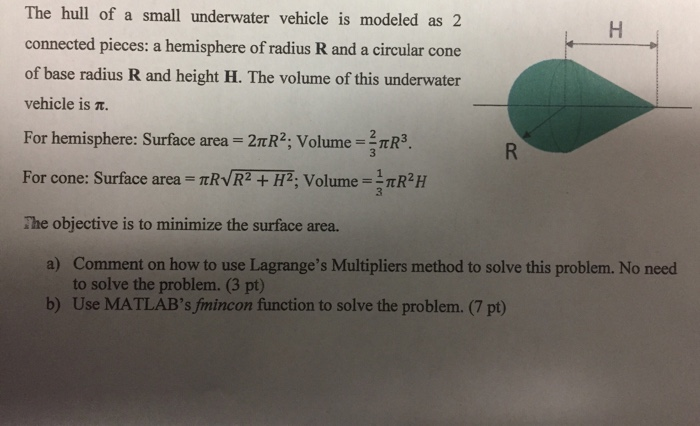In matlab The hull of a small underwater vehicle is modeled as 2 connected pieces: a hemisphere of radius R and a circular cone of base radius R and height H. The volume of this underwater vehicle is π For hemisphere: Surface area = 2πR, Volume = 3πR3. For cone. Surface area TRVR2+HP. Volume = 1 πR2H The objective is to minimize the surface area. Comment on how to use Lagrange&#39;s Multipliers method to solve this problem. No need to solve the problem. (3 pt) Use MATLAB&#39;s fmincon function to solve the problem. (7 pt) a) b)